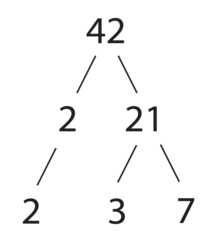# Factors Of 42 | How to Calculate Factors of 42

The factors of 42 are the integers which divide 42 exactly, without leaving a remainder. Here are the factors of 42: 1, 2, 3, 6, 7, 14, 21, 42.

You can say that factors are two different numbers that give you the original number when multiplied by each other.

Here we will talk about the factors of 42 and discuss different methods to find them. We will discuss it in-depth, so read till the end to know more about it.

## What Are The Factors Of 42?

42 is an even number which makes it easier for us to understand that there will be more numbers divisible with it. Factors of 42 are these numbers that can be divisible with 42 without leaving a remainder.

Also Check : Factors of 36

All the numbers have at least two factors; one is the number 1, while the other is the number itself. 42 has many factors other than 1 and 42. It has eight factors; the list of factors of 42 is as follows;

Positive Factors: 1, 2, 3, 6, 7, 14, 21, and 42

Negative Factors: -1, -2, -3, -6, -7, -14, -21, and -42

42 Prime Factorization: 2 × 3 × 7

Prime Factors: 2, 3, 7

Sum of Factors: 54

## Factors Of 42 In Pairs

There are two different pairs of factors, among which one is positive while the other is negative. Factors of 42 in pairs are as follow;

Positive Pair Factors of 42: (1, 42), (2, 21), (3, 14), and (6, 7)

Negative Pair Factors of 42: (-1, -42), (-2, -21), (-3, -14), and (-6, -7)

If you want to find these pair factors, then do the following step, and you'll get your pairs.

1 × 42 = 42  (1, 42)

2 × 21 = 42  (2, 21)

3 × 14 = 42  (3, 14)

6 × 7 = 42  (6, 7)

## Prime Factorization Of 42

Prime factorization is done by dividing the original number with the least prime number. In the case of 42, the least number is 1, but we don't include 1 or 42 in this method. We start with the next least number, which is 2.

In the first step, start dividing 42 with 2 which is the least prime number.

42 ÷ 2 = 21

Now in the next step, check whether the answer of the last step is divisible by 2 or not. If not, divide it by the following least number, 3. It's necessary to check this because we need a number that is divisible with it without leaving a remainder.

21 ÷ 3 = 7

Again divide it with the least number if it's possible; otherwise, go with the next divisible least number, which is 7.

7 ÷ 7 = 1

Now, you have the number 1 at the end, which indicates that you can't divide it further. 2, 3, and 7 are the prime factors of 42, we get through this method.

## Factor Tree Of 42Pic Credit: wikimedia.org

Through this factor tree, we get the same factors as we get through the Prime Factorization method. Factors of 42 through the factor tree are,

42: 2 × 3 × 7

## FAQs

### What are the divisors of 42?

1, 2, 3, 6, 7, 14, 21, and 42 are the divisors of 42.

### What are the common factors of 42?

All the common factors of 42 are as follows;

1, 2, 3, 6, 7, 14, 21, and 42.

### Write 42 as a product of prime factors.

We will get 42 when we multiply all its prime factors, which we get through the prime factorization method.

2 × 3 × 7 = 42